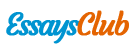# Chm420 Lab Report

Autor:   •  June 27, 2018  •  1,019 Words (5 Pages)  •  311 Views

Page 1 of 5

...

For Graham’s Law experiment, the rate diffusion for ammonia is 0.0659 cmsec-1 while for HCl is 0.0432 cmsec-1. The ratio of the rate diffusion of NH3 to the rate of diffusion of HCl obtained is 1.53. Theoretical ratio of the rates of diffusion of these gases is 1.46. Percent error for this experiment is 4.79%. Error for this experiment is using dirty instruments may affect the experiment result. So that, we should make sure all the instruments must be clean and dry before using it. Imperfections in experimental procedures also caused error for this experiment.

For molar mass of volatile liquid experiment, the molecular weight of acetone obtained is 34.426 gmol-1. Theoretical value of molecular mass for acetone is 58.08 gmol-1. Percent error for this experiment is 40.73%. Error for this experiment are the water are not boiled enough. The acetone is not fully vaporized. The analytical balance is not precise.

CONCLUSION

Ratio of the rates of diffusion of NH3 and HCl is 1.46. Molecular weight of unknown liquid (acetone) is 58.08 gmol-1

REFERENCES

- Jim Clark. (2010). Ideal Gases and the Ideal Gas Law. Retrieved from http://www.chemguide.co.uk/physical/kt/idealgases.html

QUESTIONS

-

- Rate of diffusion for NH3: 58.1cm/881sec

= 0.0659 cmsec-1

Rate of diffusion for HCl: 38.1cm/881sec

= 0.0432 cmsec-1

- 0.0659/0.0432 = 1.53

- (Rate NH3 /Rate HCl) = (Mass HCl/Mass NH3)1/2

= (36.458/17.034)1/2

= 1.46

- Percent error = Absolute value (1.46-1.53/1.46) x 100%

= 4.79%

- n = PV / RT

= 1 atm ( 0.17L) / 8.21x10-2 L atm mol-1 K-1 (338.15K)

= 0.0061 mol

Molecular weight = mass/ mol

= 0.21 / 0.0061

= 34.426 gmol-1

% error = (58.08 – 34.426/58.08) x 100%

= 40.73 %

...